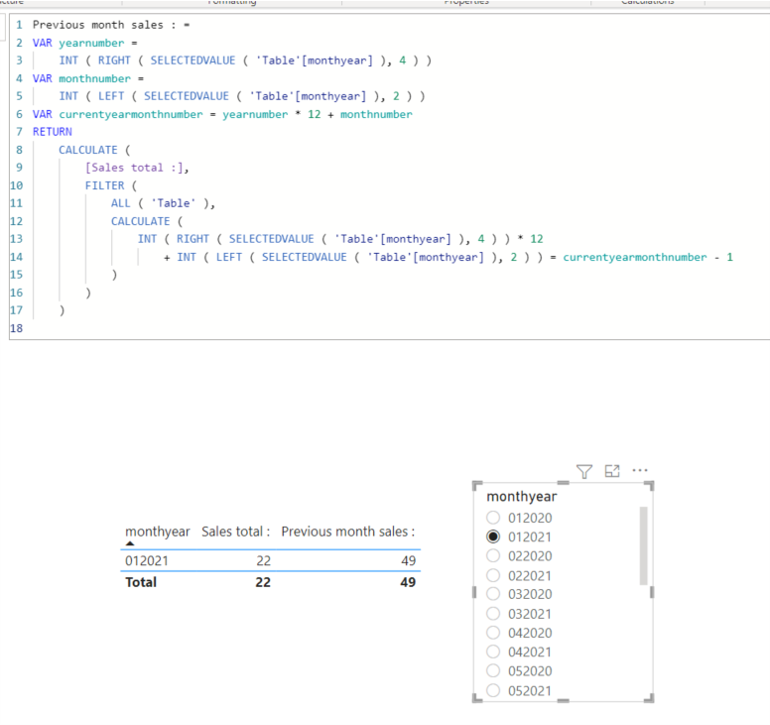cancel
Showing results for
Did you mean:Helper IV

## Getting last month sales without any date table

Hi all,

I cant use a date table for various reasons but I cant make it work how to get previous month sales without the date time intelligence.

What i want is when the user selects 2019 and month 1 he sees the sales of 2018 and month 12 next to it.

Whats the replacement for Calculate(SUM(sales), dateadd(date, month, -1) if the date table isnt there?

I have only one table with my sales and companies.

I tried using calculate(SUM(table (sales), filter(table(monthyear)= selectedvalue(monthyear) -1 but this doesnt show me nothing , just 0s. Any ideas?

Thanks!

8 REPLIES 8Super User

Having looked at your file, here's what I recommend.

Define a new calculated column:

``EoM = EOMONTH ( Data[Date], 0 )``

Then a measure:

``````PrevMonthSum =
VAR PrevMonthEnd =
EOMONTH ( SELECTEDVALUE ( Data[EoM] ), -1 )
RETURN
CALCULATE ( SUM ( Data[Amount] ), ALLSELECTED ( Data ), Data[EoM] = PrevMonthEnd )``````Helper IV

Thanks to both of you! I tried both but the problem was that the first solution @Jihwan_Kim  doesnt give me any values, I guess its because of the ALL (table) as I have to show also other entities in the table. I tried to remove that but that didnt do anything either. I only get blanks.

@AlexisOlson  When I try your  measure it gives me values but when I select month beginning like 2020 and month 1, it doesnt show the previous year month value ( it should show month 12 and year 2019) , it just shows blank. I have a filter on the page where I have monthyear when the measure works but as the user wants the date slicer in the format of Jan-2020 , then below measuer wont show me anything although the Jan-2020 is sorted by monthyear. Not sure how to resolve this?Super User

What does your [monthyear] column look like? If it's an integer like 201912, then subtracting 1 from 202001 definitely doesn't give the right result. I'd suggest working with a column that's the last day of the month so you can use EOMONTH to shift dates. You might need to create a new calculated column for this but I can't tell you quite how to write one without knowing what [monthyear] looks like.Super User

Hi,

I think the reason that my solution gives a result in my model and it does not give any result in your model is because my model and yours are different.

Without knowing your model, I can only rely on my imagination and I have to create something new.

If this post helps, then please consider accepting it as the solution to help other members find it faster, and give a big thumbs up.Helper IV

HI @Jihwan_Kim ,

I just added some dummy data where there is a date slicer int he format of aug 2021 etc and I tried your measures , but it doesnt give me any values? What am I doing wrong?

I just have the file here ; https://github.com/userdata21/test/blob/main/previous%20month.pbix

Many thanks!Super User

Hi,

Please try to fix the measure like below.

I think RIGHT function and LEFT function are missplaced.

Mine is starting with month number, but yours is starting with year number.

Previous month sales : =
VAR yearnumber =
INT ( LEFT ( SELECTEDVALUE ( Data[Sort month year] ), 4 ) )
VAR monthnumber =
INT ( RIGHT ( SELECTEDVALUE ( 'Data'[Sort month year] ), 2 ) )
VAR currentyearmonthnumber = yearnumber * 12 + monthnumber
RETURN
CALCULATE (
[Amount Sum],
FILTER (
ALL ( Data ),
CALCULATE (
INT ( LEFT ( SELECTEDVALUE ( Data[Sort month year] ), 4 ) ) * 12
+ INT ( RIGHT ( SELECTEDVALUE ( Data[Sort month year] ), 2 ) )
) = currentyearmonthnumber - 1
)
)

If this post helps, then please consider accepting it as the solution to help other members find it faster, and give a big thumbs up.Super User

Hi,

If you have a column for soring a monthyear column, it will be much easier to author DAX measure.

I assume you do not have a column for sorting a monthyear column, and monthyear column is a text type.

In that case, please check the below.Sales total : =
SUM('Table'[sales] )

Previous month sales : =
VAR yearnumber =
INT ( RIGHT ( SELECTEDVALUE ( 'Table'[monthyear] ), 4 ) )
VAR monthnumber =
INT ( LEFT ( SELECTEDVALUE ( 'Table'[monthyear] ), 2 ) )
VAR currentyearmonthnumber = yearnumber * 12 + monthnumber
RETURN
CALCULATE (
[Sales total :],
FILTER (
ALL ( 'Table' ),
CALCULATE (
INT ( RIGHT ( SELECTEDVALUE ( 'Table'[monthyear] ), 4 ) ) * 12
+ INT ( LEFT ( SELECTEDVALUE ( 'Table'[monthyear] ), 2 ) ) = currentyearmonthnumber - 1
)
)
)

If this post helps, then please consider accepting it as the solution to help other members find it faster, and give a big thumbs up.Super User

You need something to remove or replace the current month filter context.

Try something this:

``````PrevMonthSum =
VAR SelectedMonthYear = SELECTEDVALUE ( table1[monthyear] )
RETURN
CALCULATE ( SUM ( table1[sales] ), table1[monthyear] = SelectedMonthYear - 1 )``````

or this

``````SumPrevMonth =
CALCULATE (
SUM ( table1[sales] ),
FILTER (
ALL ( table1[monthyear] ),
table1[monthyear] = SELECTEDVALUE ( table1[monthyear] ) - 1
)
)``````